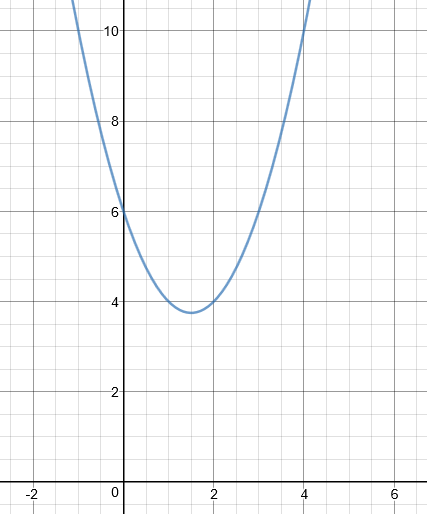# Exam-Style Questions on Graph

## Problems on Graph adapted from questions set in previous exams.

### 1.

GCSE Higher

The diagram shows part of the graph $$y=x^2-3x+6$$.(a) By drawing a suitable straight line, use your graph to find estimates for the solutions of $$x^2 - 4x + 2 = 0$$ to one decimal place.

(b) A is the point (2,4). Calculate an estimate for the gradient of the graph at the point A.

### 2.

IB Standard

Let $$f(x)=\frac{3x}{x-q}$$, where $$x \neq q$$.

(a) Write down the equations of the vertical and horizontal asymptotes of the graph of $$f$$.

The vertical and horizontal asymptotes to the graph of $$f$$ intersect at the point Q(1, 3).

(b) Find the value of q.

(c) The point P(x, y) lies on the graph of $$f$$. Show that PQ = $$\sqrt{(x-1)^2+(\frac{3}{x-1})^2}$$

(d) Hence find the coordinates of the points on the graph of $$f$$ that are closest to (1, 3).

The exam-style questions appearing on this site are based on those set in previous examinations (or sample assessment papers for future examinations) by the major examination boards. The wording, diagrams and figures used in these questions have been changed from the originals so that students can have fresh, relevant problem solving practice even if they have previously worked through the related exam paper.

The solutions to the questions on this website are only available to those who have a Transum Subscription.

Exam-Style Questions Main Page

Search for exam-style questions containing a particular word or phrase:

To search the entire Transum website use the search box in the grey area below.

Kausar Begum Khan, Kenya

Friday, January 26, 2018

"This is a fabulous website! I cannot thank you enough."

Tuesday, November 6, 2018

"Transum is so resourceful. The resources are so child-friendly that my students love its usage.It has a wide range of topics and caters to most of my needs. 'Thankyou" will be too small a word to express my gratitude to the content developers of this site."

Ahmed Jaja, Ghana

Thursday, November 22, 2018

"It was very useful for me.I recommend it to every math student.Thank you."